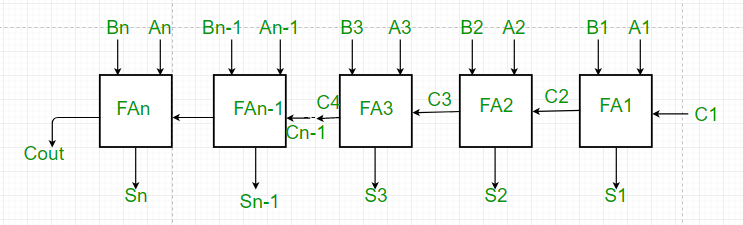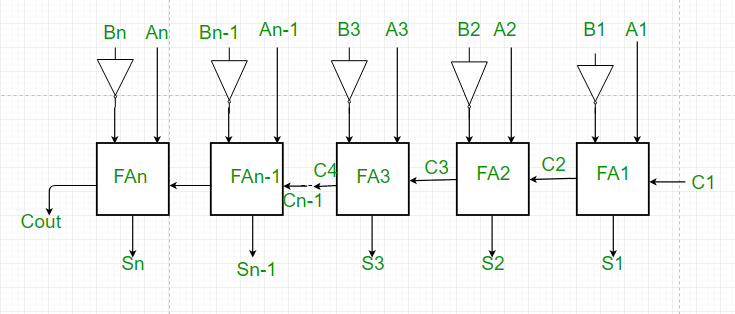# Digital Logic | Parallel Adder & Parallel Subtractor

Prerequisite – Full adder, Full Subtractor1. As shown in the figure, firstly the full adder FA1 adds A1 and B1 along with the carry C1 to generate the sum S1 (the first bit of the output sum) and the carry C2 which is connected to the next adder in chain.
2. Next, the full adder FA2 uses this carry bit C2 to add with the input bits A2 and B2 to generate the sum S2(the second bit of the output sum) and the carry C3 which is again further connected to the next adder in chain and so on.
3. The process continues till the last full adder FAn uses the carry bit Cn to add with its input An and Bn to generate the last bit of the output along last carry bit Cout.

Parallel Subtractor –

A Parallel Subtractor is a digital circuit capable of finding the arithmetic difference of two binary numbers that is greater than one bit in length by operating on corresponding pairs of bits in parallel. The parallel subtractor can be designed in several ways including combination of half and full subtractors, all full subtractors or all full adders with subtrahend complement input.Working of Parallel Subtractor –

1. As shown in the figure, the parallel binary subtractor is formed by combination of all full adders with subtrahend complement input.
2. This operation considers that the addition of minuend along with the 2’s complement of the subtrahend is equal to their subtraction.
3. Firstly the 1’s complement of B is obtained by the NOT gate and 1 can be added through the carry to find out the 2’s complement of B. This is further added to A to carry out the arithmetic subtraction.
4. The process continues till the last full adder FAn uses the carry bit Cn to add with its input An and 2’s complement of Bn to generate the last bit of the output along last carry bit Cout.

2. Time required for addition does not depend on the number of bits.
3. The output is in parallel form i.e all the bits are added/subtracted at the same time.
4. It is less costly.

1. Each adder has to wait for the carry which is to be generated from the previous adder in chain.
2. The propagation delay( delay associated with the travelling of carry bit) is found to increase with the increase in the number of bits to be added.My Personal Notes arrow_drop_upCheck out this Author's contributed articles.

If you like GeeksforGeeks and would like to contribute, you can also write an article using contribute.geeksforgeeks.org or mail your article to contribute@geeksforgeeks.org. See your article appearing on the GeeksforGeeks main page and help other Geeks.

Please Improve this article if you find anything incorrect by clicking on the "Improve Article" button below.

Article Tags :

Be the First to upvote.

Please write to us at contribute@geeksforgeeks.org to report any issue with the above content.